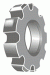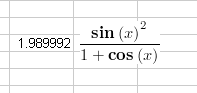•http://facebook.com/
•https://www.google.com/accounts/o8/id
•https://me.yahoo.com

# Graphical Equations for Excel (beta)18 Jul 08, 10:53PM
Graphical Equations for Excel (beta)
The Excel equation renderer has moved on a lot thanks to some great feedback.

<div class="yellowbox"> For anyone new here is a brief recap of what it does: In excel formulas that start with an '=' sign are often difficult to understand and read (especially long ones), in addition it is often nice to display the equation along side the cell to improve the documentation and explain to others who use your Excel sheet what different parts do. Our Excel equation render automatically format your equation into a graphical form making your equations easy to read. </div>

For example.

The Excel Formula:
=(SIN(x)^2)/(1+COS(x))
is rendered to give: Or if you prefer to see a screen shot:Features include:
• render any excel equation upto 220 characters long [Limitation for this first version only].
• equations rendered to the right of cell with the formula.
• cell references are rendered in blue to distinguish them from names variables.
• cell name definitions are used if entered into the formula.
• excel function names are shown in lowercase and in bold.
• render the equation with or without a border
• multiple cells may be selected and rendered at once [upto a max of 100 equations]
• move the rendered equation to any location across the document.
• re-render an equation and the original graphical will be replaced - any changes in position will be maintaining.
• select the rendered equation(s) and re-rendered them. The system will load the new formula from the same cell that defined the original rendered equation.
• you can select either the cell or the rendered equation and delete the rendered equation.
• you can delete all rendered images with a single click.

To install the latest copy, please delete all previous versions (and CodeCogs toolbars) and install the follow Excel Addin: Examples of this in use are: [To see the alpha version development comments goto: http://www.codecogs.com/pages/forums/pagegen.php?id=1008 ]